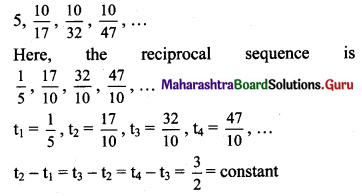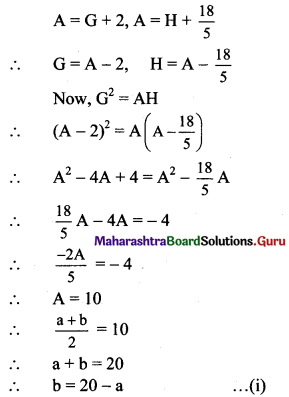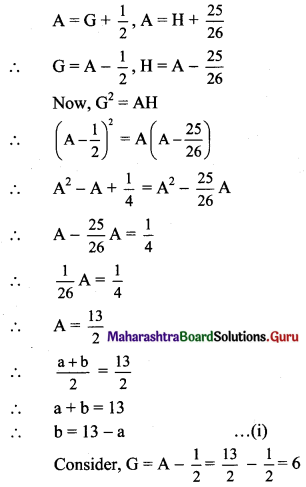# Maharashtra Board 11th Maths Solutions Chapter 2 Sequences and Series Ex 2.4

Balbharti Maharashtra State Board 11th Maths Book Solutions Pdf Chapter 2 Sequences and Series Ex 2.4 Questions and Answers.

## Maharashtra State Board 11th Maths Solutions Chapter 2 Sequences and Series Ex 2.4

Question 1.
Verify whether the following sequences are H.P.
(i) $$\frac{1}{3}, \frac{1}{5}, \frac{1}{7}, \frac{1}{9}, \ldots$$
Solution:
$$\frac{1}{3}, \frac{1}{5}, \frac{1}{7}, \frac{1}{9}, \ldots$$
Here, the reciprocal sequence is 3, 5, 7, 9,…
t1 = 3, t2 = 5, t3 = 7, t4 = 9, …..
t2 – t1 = t3 – t2 = t4 – t3 = 2 = constant
∴ The reciprocal sequence is an A.P.
∴ The given sequence is a H.P.

(ii) $$\frac{1}{3}, \frac{1}{6}, \frac{1}{12}, \frac{1}{24}, \ldots$$
Solution:
$$\frac{1}{3}, \frac{1}{6}, \frac{1}{12}, \frac{1}{24}, \ldots$$
Here, the reciprocal sequence is 3, 6, 12, 24,…
t1 = 3, t2 = 6, t3 = 12, ……
t2 – t1 = 3, t3 – t2 = 6
t2 – t1 ≠ t3 – t2
∴ The reciprocal sequence is not an A.P.
∴ The given sequence is not a H.P.(iii) $$5, \frac{10}{17}, \frac{10}{32}, \frac{10}{47}, \ldots$$
Solution:∴ The reciprocal sequence is an A.P.
∴ The given sequence is a H.P.

Question 2.
Find the nth term and hence find the 8th term of the following HPs.
(i) $$\frac{1}{2}, \frac{1}{5}, \frac{1}{8}, \frac{1}{11}, \ldots$$
Solution:
$$\frac{1}{2}, \frac{1}{5}, \frac{1}{8}, \frac{1}{11}, \ldots$$ are in H.P.
∴ 2, 5, 8, 11,… are in A.P.
∴ a = 2, d = 3
tn = a + (n – 1)d
= 2 + (n – 1)(3)
= 3n – 1
∴ nth term of H.P. = $$\frac{1}{3 n-1}$$
∴ 8th term of H.P. = $$\frac{1}{3(8)-1}$$ = $$\frac{1}{23}$$

(ii) $$\frac{1}{4}, \frac{1}{6}, \frac{1}{8}, \frac{1}{10}, \ldots$$
Solution:
$$\frac{1}{4}, \frac{1}{6}, \frac{1}{8}, \frac{1}{10}, \ldots$$ are in H.P.
∴ 4, 6, 8, 10, … are in A.P.
∴ a = 4, d = 2
tn = a + (n – 1)d
= 4 + (n – 1) (2)
= 2n + 2
∴ nth term of H.P. = $$\frac{1}{2 n+2}$$
∴ 8th term of H.P. = $$\frac{1}{2(8)+2}$$ = $$\frac{1}{18}$$

(iii) $$\frac{1}{5}, \frac{1}{10}, \frac{1}{15}, \frac{1}{20}, \ldots$$
Solution:
$$\frac{1}{5}, \frac{1}{10}, \frac{1}{15}, \frac{1}{20}, \ldots$$ are in H.P.
∴ 5, 10, 15, 20, … are in A.P.
∴ a = 5, d = 5
tn = a + (n – 1)d
= 5 + (n – 1) (5)
= 5n
∴ nth term of H.P. = $$\frac{1}{5 n}$$
∴ 8th term of H.P. = $$\frac{1}{5(8)}$$ = $$\frac{1}{40}$$Question 3.
Find A.M. of two positive numbers whose G.M. and H.M. are 4 and $$\frac{16}{5}$$ respectively.
Solution:
G.M. = 4, H.M. = $$\frac{16}{5}$$
Now, (G.M.)2 = (A.M.) (H.M.)
∴ 42 = A.M. × $$\frac{16}{5}$$
∴ A.M. = 16 × $$\frac{5}{16}$$
∴ A.M. = 5

Question 4.
Find H.M. of two positive numbers whose A.M. and G.M. are $$\frac{15}{2}$$ and 6.
Solution:
A.M. = $$\frac{15}{2}$$, G.M. = 6
Now, (G.M.)2 = (A.M.) (H.M.)
∴ 62 = $$\frac{15}{2}$$ × H.M.
∴ H.M. = 36 × $$\frac{2}{15}$$
∴ H.M. = $$\frac{24}{5}$$

Question 5.
Find G.M. of two positive numbers whose A.M. and H.M. are 75 and 48.
Solution:
A.M. = 75, H.M. = 48
Now, (G.M.)2 = (A.M.) (H.M.)
∴ (G.M.)2 = 75 × 48
∴ (G.M.)2 = 25 × 3 × 16 × 3
∴ (G.M.)2 = 52 × 42 × 32
∴ G.M. = 5 × 4 × 3
∴ G.M. = 60Question 6.
Insert two numbers between $$\frac{1}{4}$$ and $$\frac{1}{3}$$ so that the resulting sequence is a H.P.
Solution:
Let the required numbers be $$\frac{1}{\mathrm{H}_{1}}$$ and $$\frac{1}{\mathrm{H}_{2}}$$.
∴ $$\frac{1}{4}, \frac{1}{\mathrm{H}_{1}}, \frac{1}{\mathrm{H}_{2}}, \frac{1}{3}$$ are in H.P.
∴ 4, H1, H2, 3 are in A.P.
t1 = 4, t2 = H1, t3 = H2, t4 = 3
∴ t1 = a = 4, t4 = 3
tn = a + (n – 1)d
t4 = 4 + (4 – 1)d
3 = 4 + 3d
3d = -1
∴ d = $$\frac{-1}{3}$$
H1 = t2 = a + d = 4 – $$\frac{1}{3}$$ = $$\frac{11}{3}$$
H2 = t3 = a + 2d = 4 – $$\frac{2}{3}$$ = $$\frac{10}{3}$$
∴ For resulting sequence to be H.P. we need to insert numbers $$\frac{3}{11}$$ and $$\frac{3}{10}$$.

Question 7.
Insert two numbers between 1 and -27 so that the resulting sequence is a G.P.
Solution:
Let the required numbers be G1 and G2.
∴ 1, G1, G2, -27 are in G.P.
t1 = 1, t2 = G1, t3 = G2, t4 = -27
∴ t1 = a = 1
tn = arn-1
t4 = (1) r4-1
-27 = r3
r3 = (-3)3
∴ r = -3
∴ G1 = t2 = ar = 1(-3) = -3
G2 = t3 = ar2 = 1(-3)2 = 9
∴ For resulting sequence to be G.P. we need to insert numbers -3 and 9.Question 8.
If the A.M. of two numbers exceeds their G.M. by 2 and their H.M. by $$\frac{18}{5}$$, find the numbers.
Solution:
Let a and b be the two numbers.
A = $$\frac{a+b}{2}$$, G = $$\sqrt{a b}$$, H = $$\frac{2 a b}{a+b}$$
According to the given conditions,Consider, G = A – 2 = 10 – 2 = 8
$$\sqrt{a b}$$ = 8
ab = 64
a(20 – a) = 64 …..[From (i)]
a2 – 20a + 64 = 0
(a – 4)(a – 16) = 0
∴ a = 4 or a = 16
When a = 4, b = 20 – 4 = 16
When a = 16, b = 20 – 16 = 4
∴ The two numbers are 4 and 16.Question 9.
Find two numbers whose A.M. exceeds their G.M. by $$\frac{1}{2}$$ and their H.M. by $$\frac{25}{26}$$.
Solution:
Let a and b be the two numbers.
A = $$\frac{a+b}{2}$$, G = $$\sqrt{a b}$$, H = $$\frac{2 a b}{a+b}$$
According to the given conditions,$$\sqrt{a b}$$ = 6
ab = 36
a(13 – a) = 36 ……[From (i)]
a2 – 13a + 36 = 0
(a – 4)(a – 9) = 0
∴ a = 4 or a = 9
When a = 4, b = 13 – 4 = 9
When a = 9, b = 13 – 9 = 4
∴ The two numbers are 4 and 9.